Previous Page | Next Page

 The REG Procedure

## Example 74.4 Regression with Quantitative and Qualitative Variables

At times it is desirable to have independent variables in the model that are qualitative rather than quantitative. This is easily handled in a regression framework. Regression uses qualitative variables to distinguish between populations. There are two main advantages of fitting both populations in one model. You gain the ability to test for different slopes or intercepts in the populations, and more degrees of freedom are available for the analysis.

Regression with qualitative variables is different from analysis of variance and analysis of covariance. Analysis of variance uses qualitative independent variables only. Analysis of covariance uses quantitative variables in addition to the qualitative variables in order to account for correlation in the data and reduce MSE; however, the quantitative variables are not of primary interest and merely improve the precision of the analysis.

Consider the case where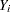is the dependent variable,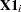is a quantitative variable,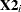is a qualitative variable taking on values 0 or 1, and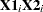is the interaction. The variableis called a dummy, binary, or indicator variable. With values 0 or 1, it distinguishes between two populations. The model is of the form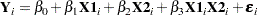for the observations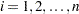. The parameters to be estimated are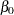,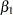,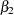, and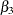. The number of dummy variables used is one less than the number of qualitative levels. This yields a nonsingular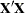matrix. See Chapter 10 of Neter, Wasserman, and Kutner (1990) for more details.

An example from Neter, Wasserman, and Kutner (1990) follows. An economist is investigating the relationship between the size of an insurance firm and the speed at which it implements new insurance innovations. He believes that the type of firm might affect this relationship and suspects that there might be some interaction between the size and type of firm. The dummy variable in the model enables the two firms to have different intercepts. The interaction term enables the firms to have different slopes as well.

In this study,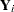is the number of months from the time the first firm implemented the innovation to the time it was implemented by theth firm. The variableis the size of the firm, measured in total assets of the firm. The variabledenotes the firm type; it is 0 if the firm is a mutual fund company and 1 if the firm is a stock company. The dummy variable enables each firm type to have a different intercept and slope.

The previous model can be broken down into a model for each firm type by plugging in the values for. If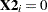, the model is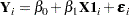This is the model for a mutual company. If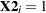, the model for a stock firm is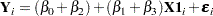This model has intercept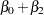and slope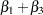.

The data1 follow. Note that the interaction term is created in the DATA step since polynomial effects such as size*type are not allowed in the MODEL statement in the REG procedure.

```title 'Regression With Quantitative and Qualitative Variables';
data insurance;
input time size type @@;
sizetype=size*type;
datalines;
17 151 0   26  92 0   21 175 0   30  31 0   22 104 0
0 277 0   12 210 0   19 120 0    4 290 0   16 238 0
28 164 1   15 272 1   11 295 1   38  68 1   31  85 1
21 224 1   20 166 1   13 305 1   30 124 1   14 246 1
;
run;
```

The following statements begin the analysis:

```proc reg data=insurance;
model time = size type sizetype;
run;
```

The ANOVA table is displayed in Output 74.4.1.

Output 74.4.1 ANOVA Table and Parameter Estimates
 Regression With Quantitative and Qualitative Variables

The REG Procedure
Model: MODEL1
Dependent Variable: time

Analysis of Variance
Source DF Sum of
Squares
Mean
Square
F Value Pr > F
Model 3 1504.41904 501.47301 45.49 <.0001
Error 16 176.38096 11.02381
Corrected Total 19 1680.80000

 Root MSE R-Square 3.32021 0.8951 19.4 0.8754 17.1145

Parameter Estimates
Variable DF Parameter
Estimate
Standard
Error
t Value Pr > |t|
Intercept 1 33.83837 2.44065 13.86 <.0001
size 1 -0.10153 0.01305 -7.78 <.0001
type 1 8.13125 3.65405 2.23 0.0408
sizetype 1 -0.00041714 0.01833 -0.02 0.9821

The overallstatistic is significant (=45.490,<0.0001). The interaction term is not significant (=0.023,=0.9821). Hence, this term should be removed and the model refitted, as shown in the following statements:

```   delete sizetype;
print;
run;
```

The DELETE statement removes the interaction term (sizetype) from the model. The new ANOVA and parameter estimates tables are shown in Output 74.4.2.

Output 74.4.2 ANOVA Table and Parameter Estimates
Analysis of Variance
Source DF Sum of
Squares
Mean
Square
F Value Pr > F
Model 2 1504.41333 752.20667 72.50 <.0001
Error 17 176.38667 10.37569
Corrected Total 19 1680.80000

 Root MSE R-Square 3.22113 0.8951 19.4 0.8827 16.6038

Parameter Estimates
Variable DF Parameter
Estimate
Standard
Error
t Value Pr > |t|
Intercept 1 33.87407 1.81386 18.68 <.0001
size 1 -0.10174 0.00889 -11.44 <.0001
type 1 8.05547 1.45911 5.52 <.0001

The overallstatistic is still significant (=72.497,<0.0001). The intercept and the coefficients associated with size and type are significantly different from zero (=18.675,<0.0001;=11.443,<0.0001;=5.521,<0.0001, respectively). Notice that thedid not change with the omission of the interaction term.

The fitted model is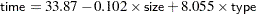The fitted model for a mutual fund company (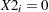) is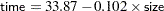and the fitted model for a stock company (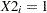) is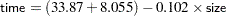So the two models have different intercepts but the same slope.

The following statements first use an OUTPUT statement to save the residuals and predicted values from the new model in the OUT= data set. Next PROC SGPLOT is used to produce Output 74.4.3, which plots residuals versus predicted values. The firm type is used as the plot symbol; this can be useful in determining if the firm types have different residual patterns.

```   output out=out r=r p=p;
run;

proc sgplot data=out;
scatter x=p y=r / markerchar=type group=type;
run;
```

Output 74.4.3 Plot of Residual vs. Predicted Values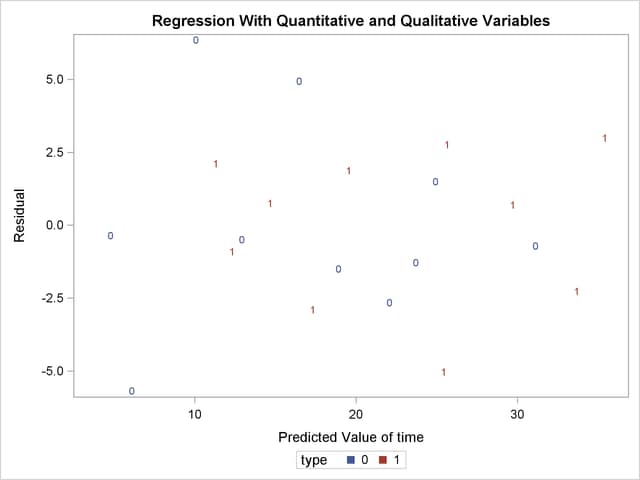The residuals show no major trend. Neither firm type by itself shows a trend either. This indicates that the model is satisfactory.

The following statements produce the plot of the predicted values versus size that appears in Output 74.4.4, where the firm type is again used as the plotting symbol:

```
proc sgplot data=out;
scatter x=size y=p / markerchar=type group=type;
run;
```

Output 74.4.4 Plot of Predicted vs. Size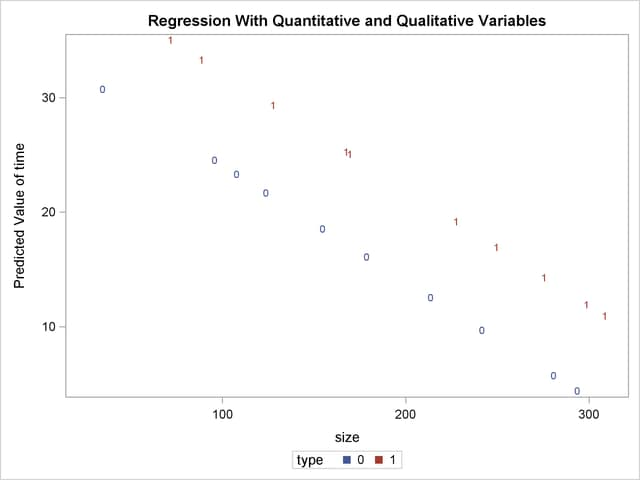The different intercepts are very evident in this plot.Previous Page | Next Page | Top of Page